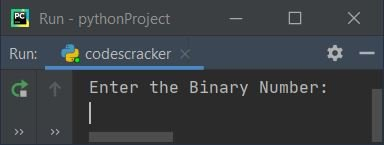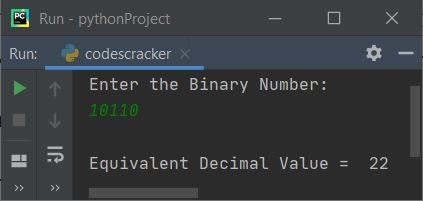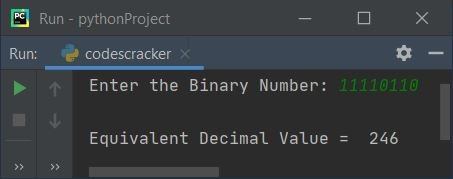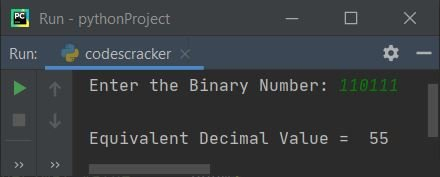# Python Program to Convert Binary to Decimal

In this article, we've created some programs in Python, to convert binary number entered by user at run-time to its equivalent decimal value. Here are the list of programs:

• Binary to Decimal using while loop
• Using for loop
• Using int()
• Using function
• Using class

Note - Before creating these programs, if you're are not aware about steps used for the conversion, refer to Binary to Decimal Conversion Steps and Formula to get every required thing about the topic.

## Binary to Decimal using while Loop

To convert binary to decimal number in Python, you have to ask from user to enter a number in binary number system to convert that number into decimal number system as shown in the program given here.

The question is, write a Python program to convert binary to decimal using while loop. Here is its answer:

```print("Enter the Binary Number: ")
bnum = int(input())

dnum = 0
i = 1
while bnum!=0:
rem = bnum%10
dnum = dnum + (rem*i)
i = i*2
bnum = int(bnum/10)

print("\nEquivalent Decimal Value = ", dnum)```

Here is its sample run. This is the initial output:Now supply the input say 10110 as binary number, press `ENTER` key to convert it into its equivalent decimal value:The dry run of following block of code:

```while bnum!=0:
rem = bnum%10
dnum = dnum + (rem*i)
i = i*2
bnum = int(bnum/10)```

with user input, 10110 goes like:

• Initial value, bnum=10110 (entered by user), dnum=0, i=1
• The condition bnum!=0 (of while loop) or 10110!=0 evaluates to be true, therefore program flow goes inside the loop and evaluates all its four statements, one by one
• That is, bnum%10 or 10110%10 or 0 gets initialized to rem. And dnum+(rem*i) or 0+(0*1) or 0 gets initialized to dnum. Then i*2 or 1*2 or 2 gets initialized to i. Finally int(bnum/10) or int(10110/10) or 1011 gets initialized to bnum
• The condition of while loop again gets evaluated with new value of bnum. That is, bnum!=0 or 1011!=0 evaluates to be true, therefore program flow again goes inside the loop
• bnum%10 or 1011%10 or 1 gets initialized to rem
• dnum+(rem*i) or 0+(1*2) or 2 gets initialized to dnum
• i*2 or 2*2 or 4 gets initialized to i
• int(bnum/10) or int(1011/10) or 101 gets initialized to bnum
• Again the condition bnum!=0 or 101!=0 evaluates to be true, therefore program flow again goes inside the loop
• bnum%10 or 101%10 or 1 gets initialized to rem
• dnum+(rem*i) or 2+(1*4) or 6 gets initialized to dnum
• i*2 or 4*2 or 8 gets initialized to i
• int(bnum/10) or int(101/10) or 10 gets initialized to bnum
• Again the condition bnum!=0 or 10!=0 evaluates to be true, therefore program flow again goes inside the loop
• bnum%10 or 10%10 or 0 gets initialized to rem
• dnum+(rem*i) or 6+(0*8) or 6 gets initialized to dnum
• i*2 or 8*2 or 16 gets initialized to i
• int(bnum/10) or int(10/10) or 1 gets initialized to bnum
• Again the condition bnum!=0 or 1!=0 evaluates to be true, therefore program flow again goes inside the loop
• bnum%10 or 1%10 or 1 gets initialized to rem
• dnum+(rem*i) or 6+(1*16) or 22 gets initialized to dnum
• i*2 or 16*2 or 32 gets initialized to i
• int(bnum/10) or int(1/10) or 0 gets initialized to bnum
• Now the condition bnum!=0 or 0!=0 evaluates to be false, therefore the execution of while loop gets ended
• And the value of dnum, that is 22 gets printed on output. Thats it

## Binary to Decimal using for Loop

This program uses for loop, instead of while. The str() method is used to convert any type of value to a string type. And the len() is used to find the length of a string. The range() returns a sequence of values from 0 to one less than the value defined as its argument. By default, each time the value gets incremented by 1.

```print("Enter the Binary Number: ", end="")
bnum = int(input())

dnum = 0
m = 1
blen = len(str(bnum))

for k in range(blen):
rem = bnum%10
dnum = dnum + (rem * m)
m = m * 2
bnum = int(bnum/10)

print("\nEquivalent Decimal Value = ", dnum)```

Here is its sample run with user input, 11110110:## Binary to Decimal using int()

This program uses int() method to convert binary to decimal. That is, we've received a value from user as input. While receiving the value using input() method, we've not specified the type.

Therefore the value entered by user, treated as a string type value. Therefore, using int(), the string type value gets converted into an integer type value of base 2, that is binary number using int(givenValue, 2) as shown in the program given below:

```print("Enter the Binary Number: ", end="")
bnum = input()

dnum = int(bnum, 2)
print("\nEquivalent Decimal Value = ", dnum)```

Here is its sample run with user input 110111 as binary number:## Binary to Decimal using Function

This program uses a user-defined function named BinToDec() to do the same job, that is converting of an binary number to its decimal equivalent.

```def BinToDec(b):
return int(b, 2)

print("Enter the Binary Number: ", end="")
bnum = input()

dnum = BinToDec(bnum)
print("\nEquivalent Decimal Value = ", dnum)```

The function, BinToDec() takes binary number as its argument, and returns its equivalent decimal value using int() with base 2

## Binary to Decimal using Class

This is the last program of this article, created using a class named CodesCracker, an object-oriented feature of Python.

```class CodesCracker:
def BinToDec(self, b):
return int(b, 2)

print("Enter the Binary Number: ", end="")
bnum = input()

ob = CodesCracker()
dnum = ob.BinToDec(bnum)
print("\nEquivalent Decimal Value = ", dnum)```

An object, ob is created of class CodesCracker to access its member function named BinToDec() using dot (.) operator. Rest of the things works like a normal function.

#### Same Program in Other Languages

Python Online Test

« Previous Program Next Program »